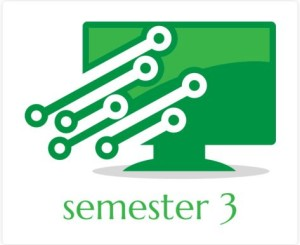# VTU CSE 3rd Sem Notes, Question Papers & Lab ProgramsIn this page you can download all the vtu cse 3rd sem materials such as vtu e-learning notes , vtu model question papers and lab programs.

#### Notes

VTU CSE 3rd sem has various subjects like Object oriented Programming , Logic design, Discrete Mathematics and so on, the vtu e learning notes for all the subjects are presented here and consists of atleast 5 to 6 units. Here students can download VTU CSE 3rd sem notes by various authors as Pdfs.

Engineering Mathematics-3 (10MAT31)

Introduction to various mathematical topics like Fourier Series, Complex Variables etc. which are covered in M3 (10MAT31).

SJBIT

Electronic Circuits (10CS32)

An introduction to electric circuit elements and electronic devices (10CS32).

SJBIT

Logic Design (10CS33)

Introduction to logic design and the basic building blocks used in digital systems, in particular digital computers (10CS33).

SJBIT

DMS (10CS34)

Introduction to Basic discrete mathematical structures: sets, relations, functions, sequences (10CS34).

SJBIT

Data Structures (10CS35)

Implementation of basic data structures like linked lists, stacks, and queues etc. covered in DS (10CS35).

SJBIT

OOPS (10CS36)

Introduction to object oriented programming with c++ or in other words, OOPS (10CS36).

SJBIT

#### Question Papers

Computer Science Sem 3 vtu model question papers of various years.  Here students can download question papers as Pdfs. Just click on the button to get these question papers. More 3rd sem model question papers will be updated soon.

#### Lab Programs

Executed lab programs of  Data Structures & Electronic Circuits. Students can access and get these executed lab programs as Pdfs.

Data Structures (10CSL37)

Electronic Circuits (10CSL38)